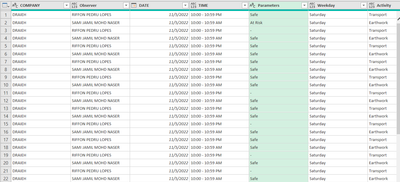cancel
Showing results for
Did you mean:Frequent Visitor

## Getting total sum per Month and get average per month per category

HI i need help on how to post calculation in power query.

i need to get the total of the Observer column per month and divide it with the total of my parameters each Safe and At risk.1 ACCEPTED SOLUTIONResolver I

netteSJ,

Starting with columns Company, Date and Parameters here is method for Power Query.

1. Transform the “Date” column to start of month and rename as “Month Starting”.
2. Add a column called “Count”, with all rows containing 1.
3. Pivot the “Parameters” column using the “Count” column as the values column.
4. Replace nulls with zeroes.
5. Group by company and start of month.
6. Add a column for total observations.
7. Add a column for percentage at risk.
8. Add a column for percentage safe.

The NaNs occur when Total Observations are zero. You may wish to filter out those rows, add some test code for zeroes or replace NaN with some text like N/A.

Here is my sample data...here is the result...and here is the M code

`let   Source = Excel.CurrentWorkbook(){[Name="Table1"]}[Content],   #"Changed Type" = Table.TransformColumnTypes(Source,      {{"Company", type text}, {"Date", type datetime}, {"Parameters", type text}}),   #"Calculated Start of Month" = Table.TransformColumns(#"Changed Type",      {{"Date", Date.StartOfMonth, type datetime}}),   #"Rename Columns to Start of Month" = Table.RenameColumns(#"Calculated Start of Month",      {{"Date", "Month Starting"}}),   #"Add Count" = Table.AddColumn(#"Rename Columns to Start of Month",       "Count",       each 1,       Int64.Type),   #"Pivot Parameters Column" = Table.Pivot(#"Add Count",       List.Distinct(#"Add Count"[Parameters]),       "Parameters",       "Count",       List.Sum),   #"Replace nulls with Zeroes" = Table.ReplaceValue(#"Pivot Parameters Column",      null,      0,      Replacer.ReplaceValue,      {"Safe", "At Risk", "-"}),   #"Group by Company and Month Starting" = Table.Group(#"Replace nulls with Zeroes",       {"Company", "Month Starting"},       {         {"Safe", each List.Sum([Safe]), type nullable number},          {"At Risk", each List.Sum([At Risk]), type nullable number}      }),   #"Add Total Observations" = Table.AddColumn(#"Group by Company and Month Starting",       "Total Observations",       each [Safe] + [At Risk],      Int64.Type),   #"Add % At Risk" = Table.AddColumn(#"Add Total Observations",       "% At Risk",       each [At Risk] / [Total Observations],       Percentage.Type),   #"Add % Safe" = Table.AddColumn(#"Add % At Risk",       "% Safe",       each [Safe] / [Total Observations],      Percentage.Type)in   #"Add % Safe"`

5 REPLIES 5Resolver I

netteSJ,

Starting with columns Company, Date and Parameters here is method for Power Query.

1. Transform the “Date” column to start of month and rename as “Month Starting”.
2. Add a column called “Count”, with all rows containing 1.
3. Pivot the “Parameters” column using the “Count” column as the values column.
4. Replace nulls with zeroes.
5. Group by company and start of month.
6. Add a column for total observations.
7. Add a column for percentage at risk.
8. Add a column for percentage safe.

The NaNs occur when Total Observations are zero. You may wish to filter out those rows, add some test code for zeroes or replace NaN with some text like N/A.

Here is my sample data...here is the result...and here is the M code

`let   Source = Excel.CurrentWorkbook(){[Name="Table1"]}[Content],   #"Changed Type" = Table.TransformColumnTypes(Source,      {{"Company", type text}, {"Date", type datetime}, {"Parameters", type text}}),   #"Calculated Start of Month" = Table.TransformColumns(#"Changed Type",      {{"Date", Date.StartOfMonth, type datetime}}),   #"Rename Columns to Start of Month" = Table.RenameColumns(#"Calculated Start of Month",      {{"Date", "Month Starting"}}),   #"Add Count" = Table.AddColumn(#"Rename Columns to Start of Month",       "Count",       each 1,       Int64.Type),   #"Pivot Parameters Column" = Table.Pivot(#"Add Count",       List.Distinct(#"Add Count"[Parameters]),       "Parameters",       "Count",       List.Sum),   #"Replace nulls with Zeroes" = Table.ReplaceValue(#"Pivot Parameters Column",      null,      0,      Replacer.ReplaceValue,      {"Safe", "At Risk", "-"}),   #"Group by Company and Month Starting" = Table.Group(#"Replace nulls with Zeroes",       {"Company", "Month Starting"},       {         {"Safe", each List.Sum([Safe]), type nullable number},          {"At Risk", each List.Sum([At Risk]), type nullable number}      }),   #"Add Total Observations" = Table.AddColumn(#"Group by Company and Month Starting",       "Total Observations",       each [Safe] + [At Risk],      Int64.Type),   #"Add % At Risk" = Table.AddColumn(#"Add Total Observations",       "% At Risk",       each [At Risk] / [Total Observations],       Percentage.Type),   #"Add % Safe" = Table.AddColumn(#"Add % At Risk",       "% Safe",       each [Safe] / [Total Observations],      Percentage.Type)in   #"Add % Safe"`Frequent VisitorResolver I

If you don't, strictly, need a solution in Power Query, the calculation you describe is ideally suited to solution in DAX + visualisation. I will illustrate the DAX solution. I loaded this dummy data into a data model.Then, I created two DAX measures:

`Count of Observations := COUNTROWS('Observations')`

and

`Fraction of Observations :=   DIVIDE(      [Count of Observations],      CALCULATE(         [Count of Observations],         REMOVEFILTERS('Observations'[Parameters])      )   )`

These measures can then be used as values in matrix visuals,Frequent Visitor

Hi Collinsg, i need to have it in Power Query. Below is data i want to have. I need to get the total Observations submitted using Column of Observer and divide it with the Total At risk and Safe to get the percentage. Which i can filter by company and month.Main Data/ Source fileSuper User

Please provide sample data that covers your issue or question completely, in a usable format (not as a screenshot).
https://community.powerbi.com/t5/Community-Blog/How-to-provide-sample-data-in-the-Power-BI-Forum/ba-...
Please show the expected outcome based on the sample data you provided.